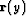Next: HEMNO: Imaging of pegleg Up: Particular Implementation of LSJIMP Previous: Differential Geometric Spreading

## Estimation/Application of Seabed Reflection Coefficient

After a pegleg multiple has been imaged, and undergone Snell Resampling and the differential geometric spreading correction, it is consistent with its primary to within the reflection coefficient of the multiple generator. In practice, the reflection coefficient always varies with space and reflection angle, though in many geologic environments these variations are both spatially smooth and small in magnitude.

In this thesis, I assume that the reflection coefficient varies in space, but does not vary with reflection angle. Assume thatandare, respectively, small windows in time, offset, and midpoint of dimension, around a primary reflection and its first pure multiple after normalized Snell Resampling and differential geometric spreading correction. The reflection coefficients,, are chosen to minimize the following quadratic functional:(25)
The second term, a finite-difference first derivative applied to the unknown, enforces a degree of smoothness across midpoint. The scalar termbalances data fitting with model smoothness. The vector of residual weights,, reflect the quality'' of the data at y.

Recall from Figurethat for a multiple and primary recorded at the same midpoint, there exists a shift in the target reflection point,, described by equation () for a first-order pegleg. In my LSJIMP implementation, variations in reflection strength of the target reflector are ignored, but not those in the multiple generator. A first justification of this assumption is convenience: the strength of the target reflection is, after all, unknown. Secondly, since the target reflection points of all legs of a pegleg are symmetric about the midpoint, the average of the reflection stregths is the same as the primary's if the true reflection strength is locally linear with midpoint.decreases with target depth, so for deep targets the local linearity assumption is likely to hold to first-order accuracy. Thirdly, ignoring target reflector variation implies that the model space of the LSJIMP inversion consists of one midpoint location only, which reduces memory usage and permits coarse-grained computer parallelization over midpoints. Therefore, when applying the reflection coefficient, we apply the coefficient at the assumed reflection point for the particular multiple being imaged. Second order multiples would be scaled by reflection coefficients from two locations, and so on.Next: HEMNO: Imaging of pegleg Up: Particular Implementation of LSJIMP Previous: Differential Geometric Spreading
Stanford Exploration Project
5/30/2004